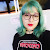# TOPIC

5% Wrong - Já refiz 4x e continua nesses 5%, help#### Ana Paula Bellon asked 1 year ago

Alguém pode me ajudar? Não faço ideia de qual seja o erro

Question solved. Code removed.

This topic was solved and cannot recieve new replies.

•#### Lizard replied 1 year ago

Eu consegui resolver, eu tava fazendo igual ao seu, mas dava o mesmo problema. Então eu criei uma nova variável com o tipo int pra imprimir os valores de 0, 1, 2, 3 , 4, 5 e os outros valores deixei em double mesmo, daí deu certo no corretor do uri. Pelo que entendi não há como representar números inteiros sendo que o valor lido era double, usando a formatação %.0lf

•#### Pereira replied 1 year ago

Ola, conseguiu solucionar? Tambem estou tendo esse problema..

•#### Elismael Menino replied 1 year ago

Conseguisse? Tb estou tendo o mesmo problema dos 5%

•#### Ana Paula Bellon replied 1 year ago

A saída:

``````
I=0 J=1
I=0 J=2
I=0 J=3
I=0.2 J=1.2
I=0.2 J=2.2
I=0.2 J=3.2
I=0.4 J=1.4
I=0.4 J=2.4
I=0.4 J=3.4
I=0.6 J=1.6
I=0.6 J=2.6
I=0.6 J=3.6
I=0.8 J=1.8
I=0.8 J=2.8
I=0.8 J=3.8
I=1 J=2
I=1 J=3
I=1 J=4
I=1.2 J=2.2
I=1.2 J=3.2
I=1.2 J=4.2
I=1.4 J=2.4
I=1.4 J=3.4
I=1.4 J=4.4
I=1.6 J=2.6
I=1.6 J=3.6
I=1.6 J=4.6
I=1.8 J=2.8
I=1.8 J=3.8
I=1.8 J=4.8
I=2 J=3
I=2 J=4
I=2 J=5
``````